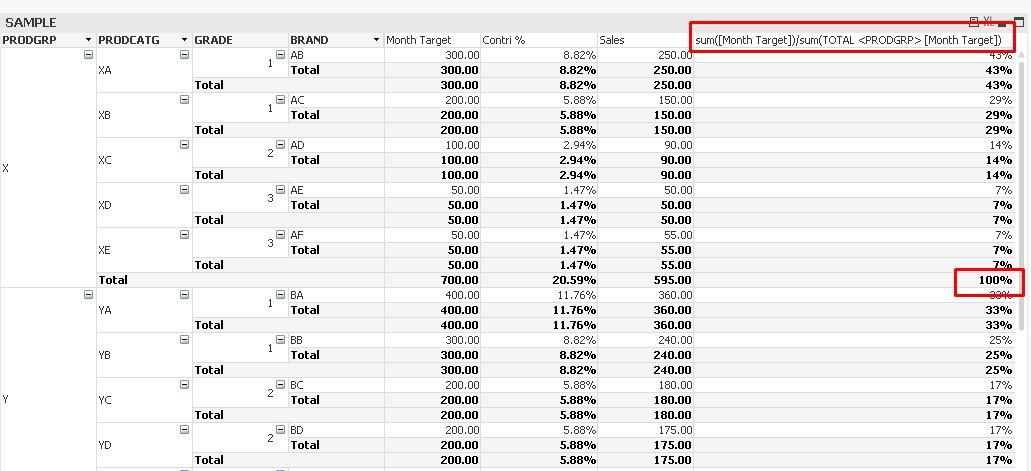# QlikView Layout & Visualizations

Discussion Board for collaboration on QlikView Layout & Visualizations.Partner

## First Dimension's Partial Sum Value used for each row percentage calculation in pivot.

Hi All,

I have a requirement from one of my Customer, in Pivot table.

There are four to five dimensions, more expressions.

First dimension is Product Group, .........

First Measure is Target Value

Next Measure is Contri %

Second measure calculation should be each row Target value / each ProductGroup's partial sum (Total) target value.

Generally we calculate as Sum(Target)/Sum( Total Target), but this wont work, because Total will be taken from Grand Total, but the requirement is each ProductGroup's total value.

Is it Possible in Qlikview pivot table?

i have attached the Sample application with this discussion.

Thanks and Regards,

Kalyan

Tags (1)
1 Solution

Accepted SolutionsMVP

## Re: First Dimension's Partial Sum Value used for each row percentage calculation in pivot.

May be like:

sum([Month Target])/sum(TOTAL <PRODGRP> [Month Target])

3 RepliesMVP

## Re: First Dimension's Partial Sum Value used for each row percentage calculation in pivot.

May be like:

sum([Month Target])/sum(TOTAL <PRODGRP> [Month Target])

Valued Contributor III

## Re: First Dimension's Partial Sum Value used for each row percentage calculation in pivot.

My solution would be the same as tresesco:Works as you wish.Partner

## Re: First Dimension's Partial Sum Value used for each row percentage calculation in pivot.

Hi Mindaugas Bacius,